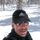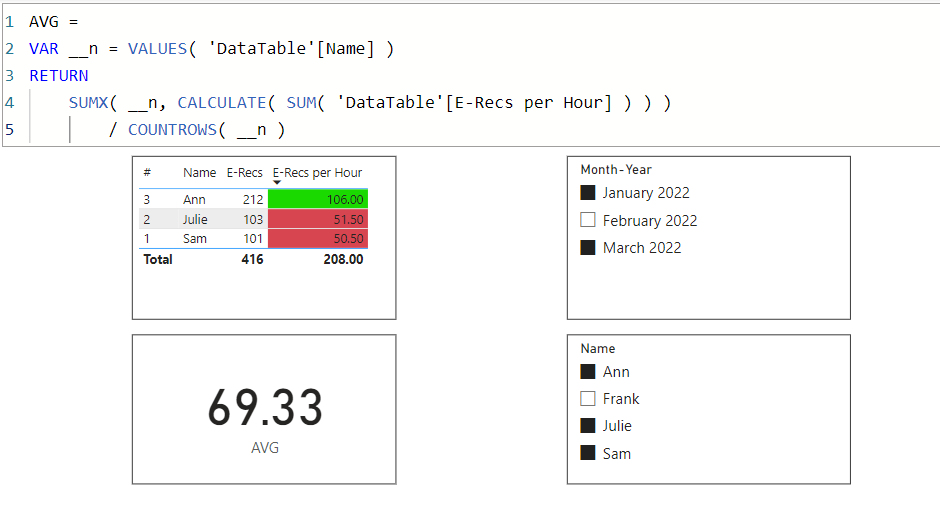cancel
Showing results for
Did you mean:Super User

## Average

Hi All,

This works with a single month selected, but not with multiple months.  Trying to show the average of what shows in the visual, and then color format the column background.Thank you!

Nathaniel

Proud to be a Super User!

3 ACCEPTED SOLUTIONSSuper User

Hi,

Could you please try the below whether it provides the result that you want?

``````Average =
AVERAGEX (
FILTER (
VALUES ( 'DataTable'[Name] ),
"@ERecsperhour", CALCULATE ( SUM ( 'DataTable'[E-Recs per Hour] ) )
),
[@ERecsperhour] <> 0
),
[@ERecsperhour]
)
``````

If this post helps, then please consider accepting it as the solution to help other members find it faster, and give a big thumbs up.Super User
``````Average = AVERAGE('DataTable'[E-Recs per Hour])
= AVERAGEX('DataTable', 'DataTable'[E-Recs per Hour])``````

the calculation of average is based on the rows of 'DataTable' in the current filter context; it has nothing to do with the calculation in the table viz.

``````AVG =
VAR __n = VALUES( 'DataTable'[Name] )
RETURN
SUMX( __n, CALCULATE( SUM( 'DataTable'[E-Recs per Hour] ) ) )
/ COUNTROWS( __n )``````Thanks to the great efforts by MS engineers to simplify syntax of DAX! Most beginners are SUCCESSFULLY MISLED to think that they could easily master DAX; but it turns out that the intricacy of the most frequently used RANKX() is still way beyond their comprehension! DAX is simple, but NOT EASY!Super User

Hi @Nathaniel_C
Here is the sample file with the solutoion https://www.dropbox.com/t/M7zX39R3UdSCO9SR

You want to calculate the average of the sum. The row level average won't help. Can follow this

``````Average New =
AVERAGEX (
VALUES ( 'DataTable'[Name] ),
CALCULATE ( SUM ( 'DataTable'[E-Recs per Hour] ) )
)``````
4 REPLIES 4Super User

Hi @Jihwan_Kim @tamerj1 @CNENFRNL ,
Thank you all,

Three different solutions, that all work!

Nathaniel

Proud to be a Super User!Super User

Hi @Nathaniel_C
Here is the sample file with the solutoion https://www.dropbox.com/t/M7zX39R3UdSCO9SR

You want to calculate the average of the sum. The row level average won't help. Can follow this

``````Average New =
AVERAGEX (
VALUES ( 'DataTable'[Name] ),
CALCULATE ( SUM ( 'DataTable'[E-Recs per Hour] ) )
)``````Super User
``````Average = AVERAGE('DataTable'[E-Recs per Hour])
= AVERAGEX('DataTable', 'DataTable'[E-Recs per Hour])``````

the calculation of average is based on the rows of 'DataTable' in the current filter context; it has nothing to do with the calculation in the table viz.

``````AVG =
VAR __n = VALUES( 'DataTable'[Name] )
RETURN
SUMX( __n, CALCULATE( SUM( 'DataTable'[E-Recs per Hour] ) ) )
/ COUNTROWS( __n )``````Thanks to the great efforts by MS engineers to simplify syntax of DAX! Most beginners are SUCCESSFULLY MISLED to think that they could easily master DAX; but it turns out that the intricacy of the most frequently used RANKX() is still way beyond their comprehension! DAX is simple, but NOT EASY!Super User

Hi,

Could you please try the below whether it provides the result that you want?

``````Average =
AVERAGEX (
FILTER (
VALUES ( 'DataTable'[Name] ),
"@ERecsperhour", CALCULATE ( SUM ( 'DataTable'[E-Recs per Hour] ) )
),
[@ERecsperhour] <> 0
),
[@ERecsperhour]
)
``````

If this post helps, then please consider accepting it as the solution to help other members find it faster, and give a big thumbs up.